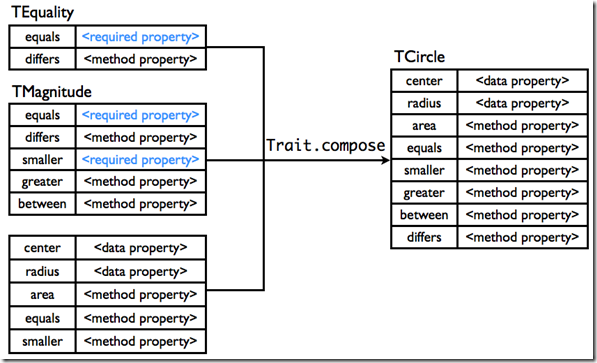# Traits in C# with Heredar

Rob Eisenberg sent me a link to a JavaScript framework named TraitsJS. I liked this example:I thought this was interesting to try using my Heredar project, so here is the code for this example:

```public interface IEquality
{
bool Equals(IEquality other);
bool Differs(IEquality other);
}

public abstract class Equality : IEquality
{
public abstract bool Equals(IEquality other);

public bool Differs(IEquality other)
{
return !Equals(other);
}
}

public interface IMagnitude : IEquality
{
bool Smaller(IMagnitude other);
bool Greater(IMagnitude other);
bool Between(IMagnitude min, IMagnitude max);
}

public abstract class Magnitude : IMagnitude
{
public abstract bool Smaller(IMagnitude other);

public abstract bool Equals(IEquality other);

public abstract bool Differs(IEquality other);

public bool Between(IMagnitude min, IMagnitude max)
{
return min.Smaller(this) && this.Smaller(max);
}

public bool Greater(IMagnitude other)
{
return !Smaller(other) && this.Differs(other);
}
}

[ExtendWith(typeof(Equality), typeof(Magnitude))]
public class Circle
{
public int Center { get; set; }

public int Radious { get; set; }

public double Area
{
get { return Math.PI * (Radious ^ 2); }
}

public bool Equals(IEquality other)
{
var otherCircle = other as Circle;
if (otherCircle == null) return false;
return otherCircle.Center == Center

}

public bool Smaller(IMagnitude other)
{
var otherCircle = other as Circle;
if (otherCircle == null) return false;
}
}```

This example uses a feature of Heredar called Abstract Templates. The weaved class looks like:

```public class Circle : IEquality, IMagnitude
{
// Fields
private Equality Equality_1;
private Magnitude Magnitude_1;

// Methods
public Circle()
{
this.Equality_1 = new EqualityImpl(this);
this.Magnitude_1 = new MagnitudeImpl(this);
}

public bool Between(IMagnitude min, IMagnitude max)
{
return this.Magnitude_1.Between(min, max);
}

public bool Differs(IEquality other)
{
return this.Equality_1.Differs(other);
}

public sealed override bool Equals(IEquality other)
{
Circle otherCircle = other as Circle;
if (otherCircle == null)
{
return false;
}
}

public bool Greater(IMagnitude other)
{
return this.Magnitude_1.Greater(other);
}

public bool Smaller(IMagnitude other)
{
Circle otherCircle = other as Circle;
if (otherCircle == null)
{
return false;
}
}

public double Area
{
get
{
return (3.1415926535897931 * (this.Radious ^ 2));
}
}

public int Center { get;  set; }

public int Radious { get; set; }

// Nested Types
private class EqualityImpl : Equality
{
// Fields
private Circle Circle;

// Methods
public EqualityImpl(Circle circle)
{
this.Circle = circle;
}

public override bool Equals(IEquality other)
{
return this.Circle.Equals(other);
}
}

private class MagnitudeImpl : Magnitude
{
// Fields
private Circle Circle;

// Methods
public MagnitudeImpl(Circle circle)
{
this.Circle = circle;
}

public override bool Differs(IEquality other)
{
return this.Circle.Differs(other);
}

public override bool Equals(IEquality other)
{
return this.Circle.Equals(other);
}

public override bool Smaller(IMagnitude other)
{
return this.Circle.Smaller(other);
}
}
}```

Note; There is a lot of cases that TraitJs supports and Heredar doesn’t, take this is as a work in progress.